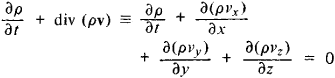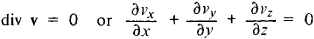# Continuity Equation

Also found in: Wikipedia.

## continuity equation

[‚känt·ən′ü·əd·ē i′kwā·zhən]
(physics)
An equation obeyed by any conserved, indestructible quantity such as mass, electric charge, thermal energy, electrical energy, or quantum-mechanical probability, which is essentially a statement that the rate of increase of the quantity in any region equals the total current flowing into the region. Also known as equation of continuity.
McGraw-Hill Dictionary of Scientific & Technical Terms, 6E, Copyright © 2003 by The McGraw-Hill Companies, Inc.
The following article is from The Great Soviet Encyclopedia (1979). It might be outdated or ideologically biased.

## Continuity Equation

one of the equations of hydrodynamics that expresses the law of the conservation of mass for any volume of moving liquid or gas. In Euler variables the continuity equation has the formwhere ρ is the density of the fluid, v is its velocity at a given point, and vx, vy, and vz are the components of the velocity along the coordinate axes. If the fluid is incompressible (ρ = const), then the continuity equation takes the formFor steady, one-dimensional flow in a pipe or channel with a cross-sectional area S, the continuity equation gives the law of constancy of the flow rate ρSv = const.

S. M. TARG

References in periodicals archive ?
As per continuity equation, the mass flow rate (product of fluid density, tube's cross-sectional area, and the fluid velocity) remains constant throughout the capillary tube.
It will be shown that in the far zone, where radiative EM fields prevail, the generalized EM energy-momentum tensor yields the standard continuity equation (Poynting theorem), hence implying equal propagation (retardation) rates for both bound and radiative EM field components.
Equation (3) is the continuity equation expressing the conservation of mass.
A continuity equation is derived for the conserved energetic field quantities averaged over the waveguide cross section.
Continuity equation, motion equation and constitutive equation are used as follows:
The topics are fluid properties, fluid statics, flowing fluids and pressure variation, the control volume approach and the continuity equation, momentum equation, the energy equation, dimensional analysis and similitude, surface resistance, flow in conduits, drag and lift, compressible flow, flow measurements, turbomachinery, and flow in open channels.
The partial differential equations (pdes) governing fluid flow and heat transfer include the continuity equation, the Navier-Stokes equations and the energy equation (Grigore &Popa, 2009).
Pressure-velocity coupling is achieved by discretisation of the continuity equation to derive an equation for pressure from the discrete continuity equation.
To derive the continuity equation of a phase, the Boltzmann equation is multiplied by the characteristic mass of the phase and is integrated over the velocity space.
The terms responsible for the gravity wave speed are the pressure gradient term in the momentum equation, g[nabla][zeta], and the divergence term in the continuity equation [nabla].
Redefining the gravitational energy density and the gravitational pressure density, the continuity equation has been satisfied.
The corresponding continuity equation of an incompressible fluid is

Site: Follow: Share:
Open / Close